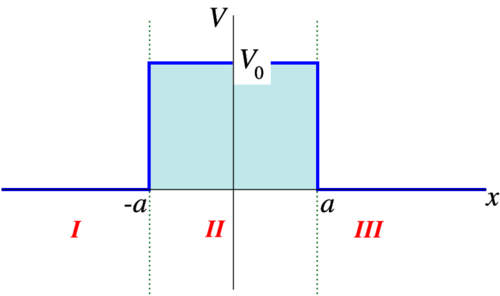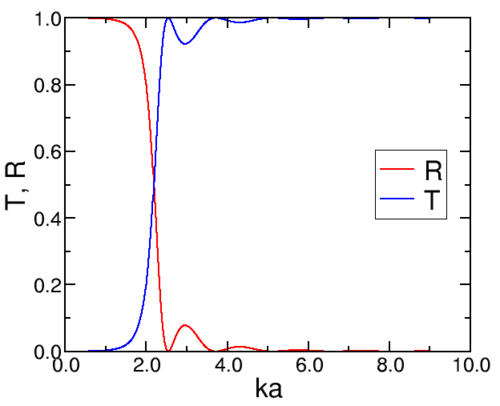# 6.3: Square Barrier

$$\newcommand{\vecs}{\overset { \rightharpoonup} {\mathbf{#1}} }$$ $$\newcommand{\vecd}{\overset{-\!-\!\rightharpoonup}{\vphantom{a}\smash {#1}}}$$$$\newcommand{\id}{\mathrm{id}}$$ $$\newcommand{\Span}{\mathrm{span}}$$ $$\newcommand{\kernel}{\mathrm{null}\,}$$ $$\newcommand{\range}{\mathrm{range}\,}$$ $$\newcommand{\RealPart}{\mathrm{Re}}$$ $$\newcommand{\ImaginaryPart}{\mathrm{Im}}$$ $$\newcommand{\Argument}{\mathrm{Arg}}$$ $$\newcommand{\norm}{\| #1 \|}$$ $$\newcommand{\inner}{\langle #1, #2 \rangle}$$ $$\newcommand{\Span}{\mathrm{span}}$$ $$\newcommand{\id}{\mathrm{id}}$$ $$\newcommand{\Span}{\mathrm{span}}$$ $$\newcommand{\kernel}{\mathrm{null}\,}$$ $$\newcommand{\range}{\mathrm{range}\,}$$ $$\newcommand{\RealPart}{\mathrm{Re}}$$ $$\newcommand{\ImaginaryPart}{\mathrm{Im}}$$ $$\newcommand{\Argument}{\mathrm{Arg}}$$ $$\newcommand{\norm}{\| #1 \|}$$ $$\newcommand{\inner}{\langle #1, #2 \rangle}$$ $$\newcommand{\Span}{\mathrm{span}}$$$$\newcommand{\AA}{\unicode[.8,0]{x212B}}$$

A slightly more involved example is the square potential barrier, an inverted square well, see Figure $$\PageIndex{1}$$.Figure $$\PageIndex{1}$$: The square barrier.

We are interested in the case that the energy is below the barrier height, $$0< E< V_0$$. If we once again assume an incoming beam of particles from the right, it is clear that the solutions in the three regions are

\begin{align} ϕ_I ( x ) &= A_1 e^{i k x} + B_1 e ^{− i k x} \\[5pt] ϕ_{II} ( x ) &= A_2 \cosh ( κ x ) + B_2 \sinh ( κ x ) \\[5pt] ϕ_{III} ( x ) &= A_3 e^{ i k x}. \label{6.16} \end{align}

Here

$k = \sqrt{\dfrac{2 m}{ℏ^2} E}$

and

$κ = \sqrt{\dfrac{2 m}{ℏ^2} ( V_0 − E )} . \label{6.17}$

Matching $$ϕ_I$$ and $$ϕ_{II}$$ at $$x=− a$$ and $$ϕ_{II}$$ and $$ϕ_{III}$$ at $$x= a$$ gives (use $$\sinh (− x)=− \sinh x$$ and $$\cosh (− x)= \cosh x$$).

\begin{align} A_1 e^{−ika} + B_1 e^{ika} &= A_2 \cosh κ a − B_2 \sinh κ a \label{6.18} \\[5pt] i k ( A_1 e^{−ika} − B_1 e^{ika} ) &= κ ( − A_2 \sinh κ a + B_2 \cosh κ a ) \label{6.19} \\[5pt] A_3 e^{ika} &= A_2 \cosh κ a + B_2 \sinh κ a \label{6.20} \\[5pt] i k ( A_3 e^{ika} ) &= κ ( A_2 \sinh κ a + B_2 \cosh κ a ) \label{6.21} \end{align}

These are four equations with five unknowns. We can thus express for of the unknown quantities in one other. Let us choose that one to be $$A_1$$, since that describes the intensity of the incoming beam. We are not interested in $$A_2$$ and $$B_2$$, which describe the wave function in the middle. We can combine the equation above so that they either have $$A_2$$ or $$B_2$$ on the right hand side, which allows us to eliminate these two variables, leading to two equations with the three interesting unknowns $$A_3$$, $$B_1$$ and $$A_1$$. These can then be solved for $$A_3$$ and $$B_1$$ in terms of $$A_1$$:

The way we proceed is to add Equations \ref{6.18} and \ref{6.20}, subtract Equations \ref{6.19} from \ref{6.21}, subtract \ref{6.20} from \ref{6.18}, and add \ref{6.19} and \ref{6.21}. We find

\begin{align} A_1 e^{−ika} + B_1 e^{ika} + A_3 e^{ika} &= 2 A_2 \cosh κ a \label{6.22} \\[5pt] i k ( − A_1 e^{−ika} + B_1 e^{ika} + A_3 e^{ika} ) &= 2 κ A_2 \sinh κ a \label{6.23} \\[5pt] A_1 e^{−ika} + B_1 e^{ika} − A_3 e^{ika} &= − 2 B_2 \sinh κ a \label{6.24} \\[5pt] i k ( A_1 e^{−ika} − B_1 e^{ika} + A_3 e^{ika} ) &= 2 κ B_2 \cosh κ a \label{6.25} \end{align}

We now take the ratio of equations \ref{6.22} and \ref{6.23} and of \ref{6.24} and \ref{6.25}, and find (i.e., we take ratios of left- and right hand sides, and equate those)

\begin{align} \dfrac{A_1 e^{−ika} + B_1 e^{ika} + A_3 e^{ika} }{i k ( − A_1 e^{−ika} + B_1 e^{ika} + A_3 e^{ika} )} &= \dfrac{1}{ κ \tanh κ a} \label{6.26} \\[5pt] \dfrac{ A_1 e^{−ika} + B_1 e^{ika} − A_3 e^{ika}}{ i k ( − A_1 e^{−ika} + B_1 e^{ika} + A_3 e^{ika} )} &= − \dfrac{\tanh κ a}{ κ} \label{6.27} \end{align}

These equations can be rewritten as (multiplying out the denominators, and collecting terms with $$A_1$$, $$B_3$$ and $$A_3$$)

\begin{align} A_1 e^{−ika} ( κ \tanh κ a + i k ) + B_1 e^{ika} ( κ \tanh κ a − i k ) + A_3 e^{ika} ( κ \tanh κ a − i k ) = 0 \label{6.28} \\[5pt] A_1 e^{−ika} ( κ − i k \tanh κ a ) + B_1 e^{ika} ( κ + i k \tanh κ a ) + A_3 e^{ika} ( − κ + i k \tanh κ a ) &= 0 \label{6.29} \end{align}

Now eliminate $$A_3$$, add Equations \ref{6.28} and \ref{6.29} to find

\begin{align} A_1 e^{−ika} [ ( κ − i k \tanh κ a ) ( κ \tanh κ a + i k ) + ( κ \tanh κ a − i k ) ( κ − i k \tanh κ a ) ] + B_1 e^{ika} [ ( κ − i k \tanh κ a ) ( κ \tanh κ a − i k ) + ( κ \tanh κ a − i k ) ( κ + i k \tanh κ a ) ] = 0 \label{6.30} \end{align}

Thus we find

$B_1 = − A_1 e ^{− 2 i k a} \dfrac{ \tanh κ a ( k^2 + κ^2 ) }{( κ − i k \tanh κ a ) ( κ \tanh κ a − i k )} \label{6.31}$

and we find, after using some of the angle-doubling formulas for hyperbolic functions, that the absolute value squared, i.e., the reﬂection coefficient, is

$R = \dfrac{ \sinh^2 2 κ a \left( κ^2 + k^2 \right)^2}{ 4 κ^2 k^2 + \left( κ^2 − k^2 \right)^2 \sinh^2 2 κ a } \label{6.32}$

In a similar way we can express $$A_3$$ in terms of $$A_1$$ (add Equations \ref{6.28} and Equation \ref{6.29}, or use

$T= 1− R!$

## Alternative approach

The equation can be given in matrix form as

$e^{− i k a} e^{ika} i k e^{− i k a} − i k e^{ika} A_1 B_1 = \cosh κ a− \sinh κ a−κ \sinh κ aκ \cosh κ a A_2 B_2 \label{6.33) e^{ika} e ^{− i k a} i k e^{ika}− i k e− i k a A_3 0 = \cosh κ a \sinh κ aκ \sinh κ aκ \cosh κ a A_2 B_2 .\label{6.34)$

Question: Can you invert the matrices and find the same answer as before?

## Example $$\PageIndex{1}$$: Hydrogen Atom Scattering

We now consider a particle of the mass of a hydrogen atom, $$m= 1.67 × 10^{−27} kg$$, and use a barrier of height 4 meV and of width $$10^{−10} m$$. The picture for reﬂection and transmission coefficients can seen in Figure $$\PageIndex{1; left}$$. We have also evaluated $$R$$ and $$T$$ for energies larger than the height of the barrier (the evaluation is straightforward).Figure $$\PageIndex{2}$$: The reﬂection and transmission coefficients for a square barrier of height 4 meV (left) amd 50 meV (right) and width 1 0− 1 0 m.

If we heighten the barrier to 50 meV, we find a slightly different picture (Figure $$\PageIndex{1; right}$$).

Notice the oscillations (resonances) in the reﬂection. These are related to an integer number of oscillations fitting exactly in the width of the barrier, $$\sin^2κ a= 0$$.

This page titled 6.3: Square Barrier is shared under a CC BY-NC-SA 2.0 license and was authored, remixed, and/or curated by Niels Walet via source content that was edited to the style and standards of the LibreTexts platform; a detailed edit history is available upon request.